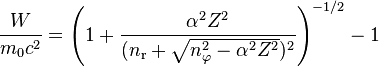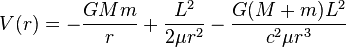# Numerical simulation the n-body problem under SR model

• A
astrof
The problem is a simple n-body problem:

there is n charged particles - electrons, protons, or other particles;
we have the initial parameters: a mass, charge, position, velocity, at a time t = 0.

In a standard (classical) numerical computation of the n-body problem I can use any numerical method based on a force, say:

F = am = k/r^2; for gravity and for the charged bodies is the same...

But I want to apply the SR model.. so, what is a difference - the force formula has other form - how is it?

The SR equation is still: E = Ek + Ep
the Ek has other form: $$E_k = mc^2(\gamma-1)$$
and the potential Ep is the same: Ep = k/r;

but what is the force which I want to apply in every step of the simulation?
F_ij = m_i ai = ... ?

Staff Emeritus
Maxell's equations are fully relativistic, so a solution of Maxwell's equations for n charged bodies will be fully relativistic. But of course n charged bodies will in general radiate, so if you want an accurate solution, you'll have to account for that. That means more degrees of freedom than an "n-body problem". I believe you'll have to solve partial differential equations rather than ordinary ones, and impose the right boundary conditions that you want at infinity.

•astrof
astrof
I use simple the second order methods for numerical computitions, ie. Verlet, RK4, ect.

My actual code for the gravitational n-body problem looks like this:

void force(TP3d *acc, const TBody *y, int n)
{
const TBody *e = y + n-1; // y is a bodies vector: r, v, m, ect. for the n bodies.
zero(acc); // acc[1..n] = 0, finally it's the accel. of the bodies

for(const TBody *p = y; p < e; p++, acc++)
{

const TBody *q = p; TP3d *a = acc;

do{ q++; a++;
TP4d r; r.sub(p->r, q->r); // TP4d is a simply 4-dim vector: r = x,y,z,s
r.s = r*r; r.s = 1.0 / (r.s*sqrt(r.s)); // s = 1/|r|^3

o->subm(r, q->m*r.s); // - r * m/r^3 => the standard Newton..

}while( q < e );
}
}

OK. Now I can use this force in any method of integration, for example a simple Euler is:

force(a, y, n);
v += a*h; // h = dt = a time step
r += v*h;

and for the RK4 it's quite simillar:

r += (h/6)*(v1 + 2*v2 + 2*v3 + v4);
v += (h/6)*(a1 + 2*a2 + 2*a3 + a4);

where the a1, a2, ... are computed at some mid-points using the same procedure: force.
...

So, what I must to modify in the force procedure to get the SR - relativistic correct version?
And the system is stationary, stable - without any loss of energy, thus no radiation!

Last edited:
Staff Emeritus
I can't say I follow the code at all. I also noticed that you've switched the topic from discussing electromagnetism to gravity mid-stream - more on that later. Considering the simpler electromagnetic case first, it's unclear to me from your code if you've included the magnetic force as well as the columb electrostatic 1/r^2 force. I see some functions called "force", but I don't see the guts of these functions in my quick look that give the force law. Additionally, I see no way that your code can possibly compute the emitted electromagnetic field and electromagnetic radiation for the electromagnetic case. This is important because the electromagnetic field can carry momentum, and the approach you used doesn't appear to account for it, assuming an instantaneous "force at a distance", rather than the actual situation in which both the particles and the fields may contain energy and momentum. The fix to include the electromagnetic field won't be a simple, I believe it will require you to reconceptualize the problem and compute not just a discreete system with n-bodies each of which has a position (which has first and second derivatives with respect to time), but rather to compute an elelctromagnetic field that fills space. To model this field, I believe you'll need much more degrees of freedom, and the resulting problem will be one that involves solving partial differential equations, not ordinary differential equations. I imagine a good E&M text like Griffiths would give you some insight, I can't say I know exactly what's needed, and I have a nagging memory that it may be even more difficult than I'm making it out to be - I recall there were some thorny issues with point charges and self-forces in classical E&M, for instance.

Going on to the gravitational case, which is considerably more complex. While special relativity is sufficient to handle the electromagnetic case, it won't be sufficient to handle the gravitational case. The view of gravity as a force is simply too limiting, you'll need a dynamic model of curved space-time, you'd need to seriously study GR before you could even begin to undertake such an effort.

•astrof
astrof
I understand these your objections, but there is the famous and successful model of the e-m interactions:
the Sommerfeld model.
Sommerfeld used the relativistic energy/mass: Ek = ..., in place of the simple: 1/2 mv^2, and he get perfectly correct results...

Dirac' solution gives exactly the same results:
https://en.wikipedia.org/wiki/Old_quantum_theory"This solution (using substitutions for quantum numbers) is equivalent to the solution of the Dirac equation".

Thus my question can be: how to simulate numerically the Sommerfeld model?

Staff Emeritus
Do you want a classical solution or a quantum solution?

astrof
I'm interested in the correct numerical solution, ie. the correct approximation,
in a practical sense... for example: in the simulation I want to see the relativistic precession,
and other effects, which are measurable in the experiments.

The quantum model is rather too primitive... for my numerical simulations,
because it's applicable for very special configurations only - de Broglie condition, etc.

Maybe I show a simpler example: the precession of the Mercury's apse.
http://en.wikipedia.org/wiki/Two-body_problem_in_general_relativity

there is a simple correction of the potential only:And I can use this to compute the force correction, which is very simply:
dg/g = 3 v^2/c^2;

I can apply this simple correction directly into my force procedure
and the relativistic apsidal precession would be correct - no?

Last edited:
Homework Helper
Gold Member
•astrof
Mentor
the Sommerfeld model

This is not a model of "the e-m interactions" in general. It's a model of the specific case of an electron in an atom. And the answers it gives are not completely correct; there are a number of effects detected by later experiments that this model does not predict.

astrof
I'm very sorry, probably together with Sommerfeld, but the old solutions of the Sommerfeld model are still the same and fully correct;
Therefore any further model must provide exactly the same results!
And despite of the greatly.. overmanipulated/improvised procedures and fantastic at hoc interpretations.

BTW. The Lamb shift and other miniscule effects has been never computed
under the Sommerfeld model in fact, thus any further claims about
the incorrectness of the model are groundless.

Last edited:
Mentor
the old solutions of the Sommerfeld model are still the same and fully correct

Please give an example of a scenario for which the old Sommerfeld model gives the exact correct answer. Note that this is not the same as the Sommerfeld model giving an acceptable approximation; you said "fully correct" so you need to justify "fully correct", not "approximately correct".

The Lamb shift and other miniscule effects has been never computed
under the Sommerfeld model in fact, thus any further claims about
the incorrectness of the model are groundless.

No, claims about the incorrectness of the model are entirely justified. When we discover new effects that an old model doesn't include, that means the old model is incorrect. It doesn't mean we ignore the new effects because the old model didn't compute them.

Staff Emeritus
This OP has his answer. He doesn't like it, but he has it. Maybe it's time to close the thread?

astrof
I'm not interested in a theoretical nor historical dispute, but if you need it, then you get that.

Please give an example of a scenario for which the old Sommerfeld model gives the exact correct answer. Note that this is not the same as the Sommerfeld model giving an acceptable approximation; you said "fully correct" so you need to justify "fully correct", not "approximately correct".

The "fully correct" means: consistent with the experimental data, ie. there has been no any contradiction noticed, till today.

No, claims about the incorrectness of the model are entirely justified. When we discover new effects that an old model doesn't include, that means the old model is incorrect. It doesn't mean we ignore the new effects because the old model didn't compute them.

I don't know nothing about any new effects incompatible with the Sommerfeld... show me them.

The situation is rather quite opposite.
Look at this:
http://en.wikipedia.org/wiki/Aufbau_principle
"In the old quantum theory prior to quantum mechanics, electrons were supposed to occupy classical elliptical orbits. The orbits with the highest angular momentum are 'circular orbits' outside the inner electrons, but orbits with low angular momentum (s- and p-orbitals) have high orbital eccentricity, so that they get closer to the nucleus and feel on average a less strongly screened nuclear charge."

So, the Somerfield still wins this play.

Mentor
I don't know nothing about any new effects incompatible with the Sommerfeld... show me them.

Hyperfine splitting of spectral lines. The anomalous Zeeman effect. The Lamb shift. That's just off the top of my head.

astrof
Sorry, but the hyperfine spplitting is just the main Sommerfield model discovery, further confirmed experimmentaly!
Thus any further model must reproduce the same splitting... unconditionally, exactly! - like a Dirac model does.

An anomalous Zeeman effect?
Nothing such existed in the classical theories... maybe in the new QM
artificial models there are some problems with a simple magnetic/motional interactions.

Last edited:
Mentor
he hyperfine spplitting is just the main Sommerfield model discovery

No, the fine structure splitting is what was accounted for in the Sommerfeld model:

https://en.wikipedia.org/wiki/Fine_structure

The hyperfine splitting was a later discovery that that model does not account for:

https://en.wikipedia.org/wiki/Hyperfine_structure

An anomalous Zeeman effect?

See, for example, here:

http://www.nyu.edu/classes/tuckerman/quant.mech/lectures/lecture_5/node3.html

astrof
Yes. The hyperfine is perfect in the Sommerfield model only!
It's very simple and stight result in this model.. only the further models have very strong problem to reproduce this effect.

Simply: the relativistic circular orbit has a little different energy than the eliptical.
And the measured energy differences are just exactly, what Sommerfeld computed long ago!

The Zeeman effect is a quite silimmilar story... just a fantastic models have a qreat problem to reproduce the simple things!

Mentor
•